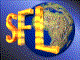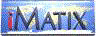| iMatix home page | << | < | > | >>SFLVersion 2.11

### http_escape

```#include "sflhttp.h"
char *
http_escape (
const char *string,
char *result,
size_t outmax)
```

#### Synopsis

Performs HTTP escaping on a string. This works as follows: all characters except alphanumerics and spaces are converted into the 3-byte sequence "%xx" where xx is the character's hexadecimal value; spaces are replaced by '+'. Line breaks are stored as "%0D%0A", where a 'line break' is any one of: "\n", "\r", "\n\r", or "\r\n". If the result buffer is NULL, calculates the required size, allocates a block of memory, and returns that. Otherwise, returns result, which must be large enough for the escaping operation (see http escape size()). When you all http escape() with a null target block, you must free the returned block using mem_free(). Returns NULL if it could not allocate a target block as required. If outmax is non-zero then no more than outmax characters (including the NULL terminator) are stored.

#### Source Code - (sflhttp.c)

```{
char
*target;                        /*  Where we store the result        */
size_t
length;                         /*  of escaped character             */

ASSERT (string);
if (outmax == 0)                    /*  If no fixed length, get total len*/
outmax = http escape size (string);

if (result == NULL)
if ((result = mem_alloc (outmax)) == NULL)
return (NULL);              /*  Could not allocate a block       */

if (outmax > 1)
outmax -= 1;                    /*  Leave space for NULL terminator  */
else
if (outmax == 1)                    /*  Only room for terminator         */
{
*result = '\0';
return (result);
}
target = result;
while (*string)
{
length = http_escape_char (*string, target, outmax, FALSE);
if (length == 0)
break;

target += length;
outmax -= length;
if (*string == '\n' || *string == '\r')
{
if ((string  == '\n' || string  == '\r')
&&  (string  != *string))
string++;
}
string++;
}
*target = '\0';                     /*  Terminate target string          */
return (result);
}
```

 | << | < | > | >>Copyright © 1996-2000 iMatix Corporation